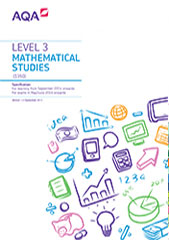These descriptions give a general indication of the level of attainment characteristic of each grade. These descriptions should be interpreted in relation to the content outlined in the Subject Content section of this specification; they are not designed to define that content.

The grade awarded will depend on how well the student has met the assessment objectives (see the Scheme of Assessment section). If a student has performed less well in some areas, this may be balanced by better performances in others.

## Level 3 Certificate Mathematical Studies

Within the context of Level 3 Certificate Mathematical Studies, students demonstrate a good understanding and knowledge of the mathematical facts, concepts and techniques that are needed, drawing on the full range of defined and assumed content to carry out set tasks successfully.

Students manipulate mathematical expressions and use graphs, sketches, tables and diagrams, all with high accuracy and skill. They use mathematical language and symbols correctly and effectively in representing situations mathematically. When confronted with unstructured problems, they can often devise and implement an effective solution strategy, communicating it appropriately and effectively. If errors are made in their calculations or logic, these are sometimes noticed and corrected.

Students recall or recognise almost all the standard models and techniques that are needed, and select appropriate ones to represent a wide variety of situations in the real world. They correctly refer results from calculations using the model to the original situation; they give sensible interpretation of their results in the context of the original situation. Their responses include mathematical justifications, explaining their solutions to problems involving a number of features or variables. They make intelligent comments on the modelling assumptions and suggest possible refinements to the model.

Students understand how almost all situations presented in the examination may be translated into mathematics. They correctly refer the results of calculations back to the given context and usually make sensible comments or predictions. They can distil the essential mathematical requirements from given data or other mathematical information.

Students make appropriate and efficient use of contemporary calculator technology and other permitted resources, and are aware of any limitations to their use. They present results to an appropriate degree of accuracy without prompting.